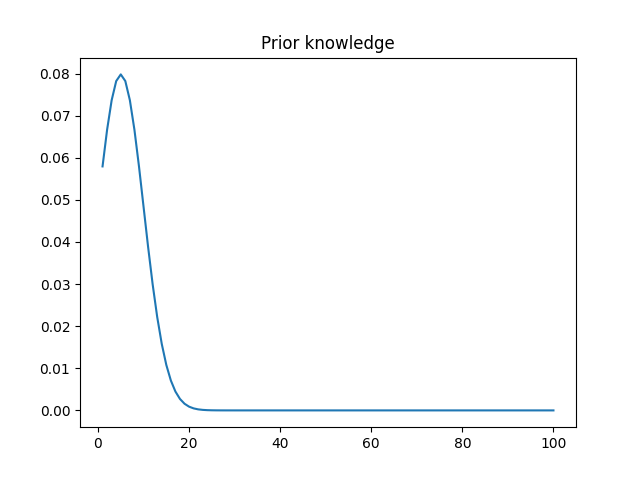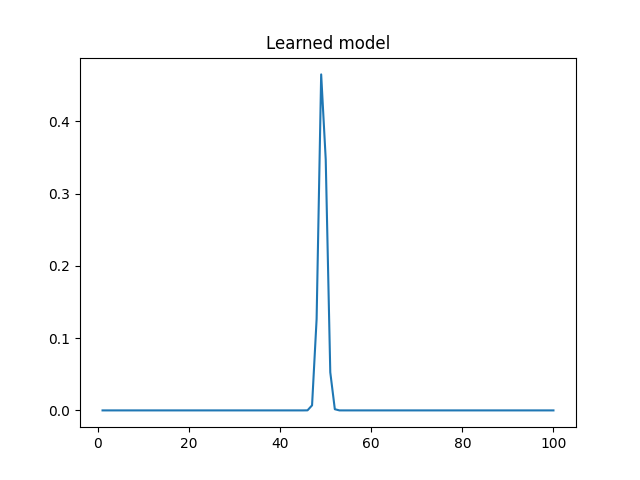# Questions on applying SVI to a normal distribution

Hello. I’m interested in using the SVI model+guide approach to model a normal distribution given some priors (in this case a normal distribution of mean 5 and stdev 5) and some observations (in this case the values 10 and 90).

This is how my prior’s PDF looks like:And this is the PDF learned by my code (mean 49.3, stdev 0.79):My questions are:

1. Why don’t I get a larger stdev? That really doesn’t look like a distribution that generated 10 and 90
2. How can I learn a model that is based on both the priors and the observations? My reasoning is that I expect the prior distribution to have significant value, but I’d like to shift it towards the observations. Similarly to a coin that I expect to be fair, but that might be biased. If I get three tails, I’m not gonna say it’s biased, but if I get 10 more tails, then it’s probably biased.

This is my code:

``````import matplotlib.pyplot as plt
import numpy as np
import pyro
from pyro.distributions import LogNormal, Normal
from pyro.infer import SVI, Trace_ELBO
import torch
from torch.distributions import constraints

pyro.enable_validation(True)
pyro.clear_param_store()
pyro.set_rng_seed(42)

data = torch.tensor([10, 90])

def model(data):
mean = torch.tensor(5.0)
stdev = torch.tensor(5.0)
payday = pyro.sample('m0', Normal(mean, stdev))

with pyro.iarange('data_loop', len(data)):
pyro.sample('m0_decoy', Normal(payday, 0.001), obs=data)

def guide(data):
mean = pyro.param('mean', torch.tensor(5.0))
stdev = pyro.param('stdev', torch.tensor(5.0), \
constraint=constraints.positive)
pyro.sample('m0', Normal(mean, stdev))

def plot(data, mean, stdev):
fig = plt.figure()
x_range = torch.tensor(np.linspace(1, 100, num=100))
y = np.exp(Normal(mean.data, stdev.data).log_prob(x_range))
plt.title('Learned model')
plt.plot(x_range, y)
plt.show()

elbo = Trace_ELBO()
svi = SVI(model, guide, optim, loss=elbo)

for i in range(1001):
svi.step(data)

if i % 50 == 0:
mean = pyro.param('mean')
stdev = pyro.param('stdev')

print("mean: {}".format(mean))
print("stdev: {}".format(stdev))

pdf = plot(data, mean, stdev)``````

Regarding my first question, I don’t know the answer, but I managed another implementation which gives me a correctly fitted normal distribution (mean 50, stdev 40) given the data (code below).

I am still interested in some ideas regarding my second question: if there’s any way to incorporate the prior knowledge in the final model, similarly to the coin toss example I mentioned earlier. I expect the coin to be fair, but it might be biased. If I get three tails, I’m not gonna say it’s biased, but if I get 10 more tails, then it’s probably biased.

``````import matplotlib.pyplot as plt
import numpy as np
import pyro
from pyro import poutine
from pyro.contrib.autoguide import AutoDelta
from pyro.distributions import Normal
from pyro.infer import SVI, config_enumerate, TraceEnum_ELBO
import torch
from torch.distributions import constraints

pyro.enable_validation(True)
pyro.clear_param_store()
pyro.set_rng_seed(42)

data = torch.tensor([10, 90])

def model(data):
mean = pyro.param('mean', torch.tensor(5.0))
stdev = pyro.param('stdev', torch.tensor(5.0), \
constraint=constraints.greater_than(0))

with pyro.iarange('data_loop', len(data)):
pyro.sample('m0', Normal(mean, stdev), obs=data)

def guide(data):
pass

def plot(data, mean, stdev):
fig = plt.figure()
x_range = torch.tensor(np.linspace(1, 100, num=100))
y = np.exp(Normal(mean.data, stdev.data).log_prob(x_range))
plt.title('Learned model')
plt.plot(x_range, y)
plt.show()

global_guide = AutoDelta(poutine.block(model, expose=['mean', 'stdev']))
optim = pyro.optim.Adam({'lr': 0.5, 'betas': [0.8, 0.99]})
elbo = TraceEnum_ELBO(max_iarange_nesting=1)
svi = SVI(model, guide, optim, loss=elbo)
pyro.param('auto_mean', torch.tensor(5.0))
pyro.param('auto_stdev', torch.tensor(5.0), \
constraint=constraints.greater_than(0))

for i in range(1001):
svi.step(data)

if i % 50 == 0:
mean = pyro.param('mean')
stdev = pyro.param('stdev')

print("mean: {}".format(mean))
print("stdev: {}".format(stdev))

plot(data, mean, stdev)``````

Solved my own problem. For future reference, code below:

``````import matplotlib.pyplot as plt
import numpy as np
import pyro
from pyro.distributions import Normal, HalfCauchy, Chi2
from pyro.infer import SVI, config_enumerate, TraceEnum_ELBO
import torch
from torch.distributions import constraints

pyro.enable_validation(True)
pyro.clear_param_store()
pyro.set_rng_seed(42)

tt = torch.tensor
data = tt([10, 90])

def model(data):
mean = pyro.sample('mean', Normal(tt(5.0), tt(10.0)))
stdev = pyro.sample('stdev', HalfCauchy(tt(1.)))

with pyro.plate('plate', len(data)):
pyro.sample('m0', Normal(mean, stdev), obs=data)

def guide(data):
var_mean = pyro.param('var_mean', tt(5.0))
var_mean_stdev = pyro.param('var_mean_stdev', tt(2.), \
constraint=constraints.positive)
var_stdev = pyro.param('var_stdev', tt(2.))

pyro.sample('mean', Normal(var_mean, var_mean_stdev))
pyro.sample('stdev', Chi2(var_stdev))

def plot(data, mean, stdev):
fig = plt.figure()
x_range = tt(np.linspace(1, 100, num=100))
y = np.exp(Normal(mean.data, stdev.data).log_prob(x_range))
plt.title('Learned model')
plt.plot(x_range, y)
plt.show()

optim = pyro.optim.Adam({'lr': 0.5, 'betas': [0.8, 0.99]})
elbo = TraceEnum_ELBO(max_iarange_nesting=1)
svi = SVI(model, guide, optim, loss=elbo)

for i in range(3001):
svi.step(data)

if i % 50 == 0:
mean = pyro.param('var_mean')
stdev = pyro.param('var_stdev')

print("mean: {}".format(mean))
print("stdev: {}".format(stdev))

plot(data, mean, stdev)``````
2 Likes